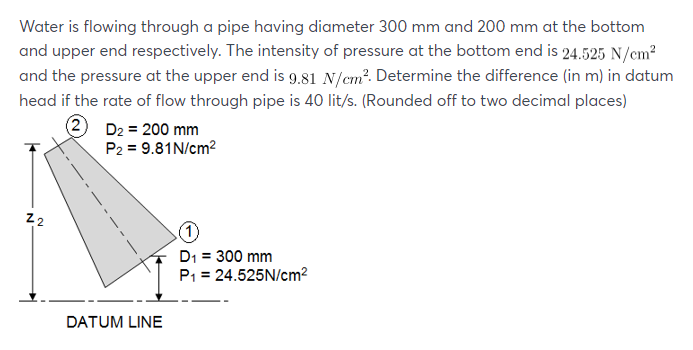# Water is flowing through a pipe having diameter 300 mm and 200 mm at the bottom end is 24.525 N/cm2 and the pressure at the upper end is 9.81 N/Cm2 . Determine the difference in datum head if the rate of flow through pipe is 40 lit/s.

Question-AnswerCategory: Fluid MechanicsWater is flowing through a pipe having diameter 300 mm and 200 mm at the bottom end is 24.525 N/cm2 and the pressure at the upper end is 9.81 N/Cm2 . Determine the difference in datum head if the rate of flow through pipe is 40 lit/s.

Water is flowing through a pipe having diameter 300 mm and 200 mm at the bottom end is 24.525 N/cm2 and the pressure at the upper end is 9.81 N/Cm2. Determine the difference in datum head if the rate of flow through pipe is 40 lit/s.Water is flowing through a pipe having diameter 300 mm and 200 mm at the bottom end is 24.525 N/cm2 and the pressure at the upper end is 9.81 N/Cm2 .Determine the difference in datum head if the rate of flow through pipe is 40 lit/s.